# LOG#069. CP(n), spheres, 1836.Data: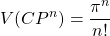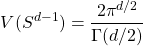where we take the radius of the sphere equal to 1 without loss of generality.

Thus,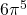is 6!=720 times the volume of the complex projective space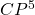. I have not found any other (simpler) way to remember that number ( it is interesting since it is really close to the proton to electron mass ratio).

We can also find that number with ratios between (hyper)-spherical volumes. There are many (indeed infinite) solutions. The ratio between the volume of a d-sphere and a d’-sphere (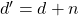and) is equal to: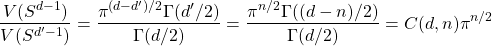We want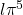, so we fixthere: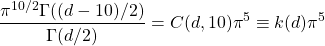and where we have defined the dimensional dependent coefficients (note that we can write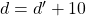, and, or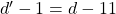)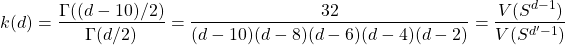I can obtain some numbers very easily: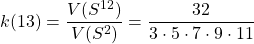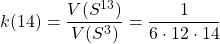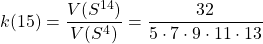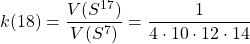I stop here sinceis the last parallelizable sphere. We get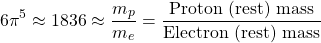as the ratio between the volumes of the following spheres:

1)times the ratio of the 10-sphere and the 0-sphere volumes, k(11).

2)times the ratio of 11-sphere and the 1-sphere (the circle) volumes, k(12).

3)times the ratio of 12-sphere and the 2-sphere  volumes, k(13).

4)times the ratio of 13-sphere and the 3-sphere volumes, k(14).

5)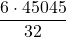times the ratio of 14-sphere and the 4-sphere  volumes, k(15).

6)times the ratio of 15-sphere and the 5-sphere  volumes, k(16).

7)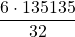times the ratio of 16-sphere and the 6-sphere volumes, k(17).

8)times the ratio of 17-sphere and the 7-sphere volumes, k(18).

9), 6! times the volume of the 5D complex projective space.

(1)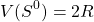(2)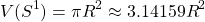(3)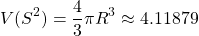(4)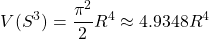(5)(6)(7)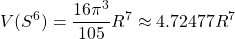(8)(9)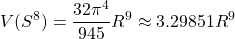(10)(11)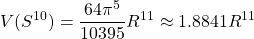(12)(13)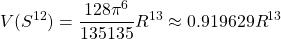(14)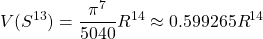(15)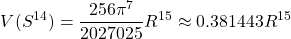(16)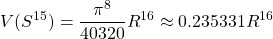(17)(18)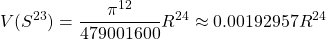(19)(20)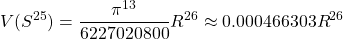(21)(22)And finally, two more…The 4096-dimensional sphere

(23)with a fantastic fraction that can not be written in the margin or space of this page easily. The final one, surprisingly, the infinite-dimensional sphere volume is zero:

(24)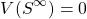The amazing vanishing sphere volume with increasing dimensions!!!!!!!!!!

PS: Made by hand and the only use of my brains and head. No common calculators but only software helped me. I am obsolete, amn’t I?

This site uses Akismet to reduce spam. Learn how your comment data is processed.